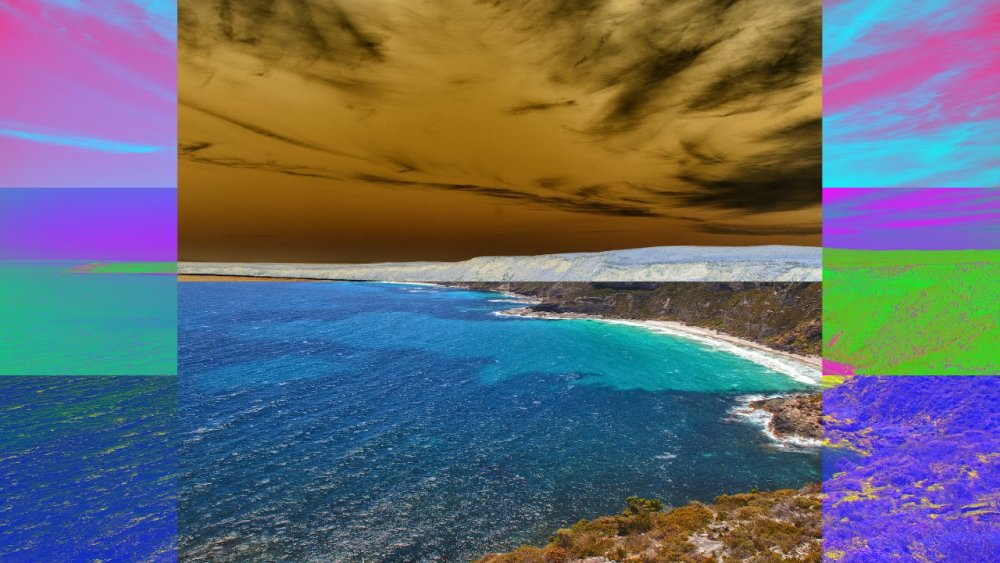## Method

Blend Modes control how images are blended together and overlayed.

These two images are the two images used for the composited examples below.

Image Input A Image Input BThis is the original image. This is the image being applied. Note that the left side is a mirror of the right, but with 50% opacity.
Blend Mode Description Example Image
Composite Image B is placed over Image A. Only available within the Composite Sources node.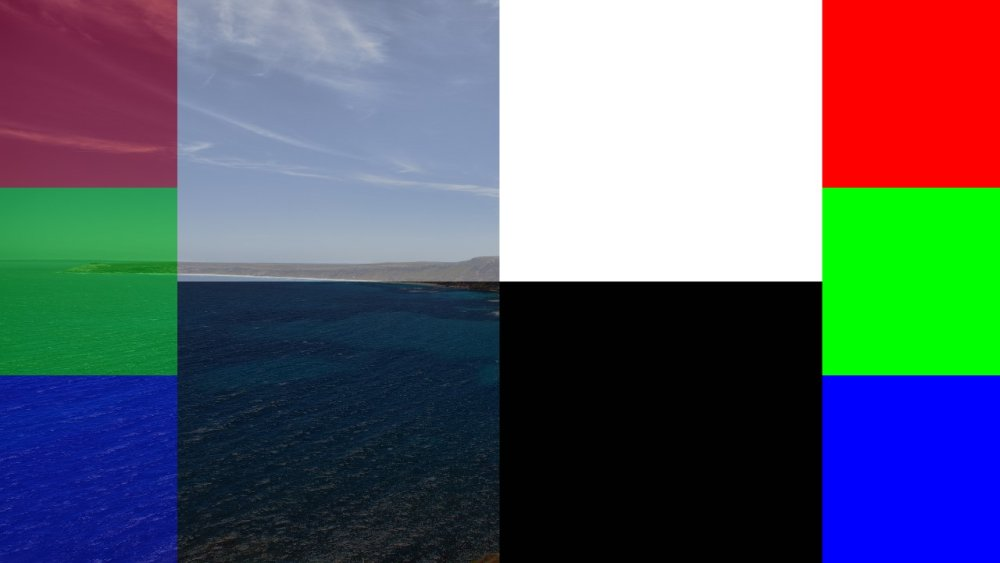Multiply Multiplies the values of image A and B together to produce a darker image.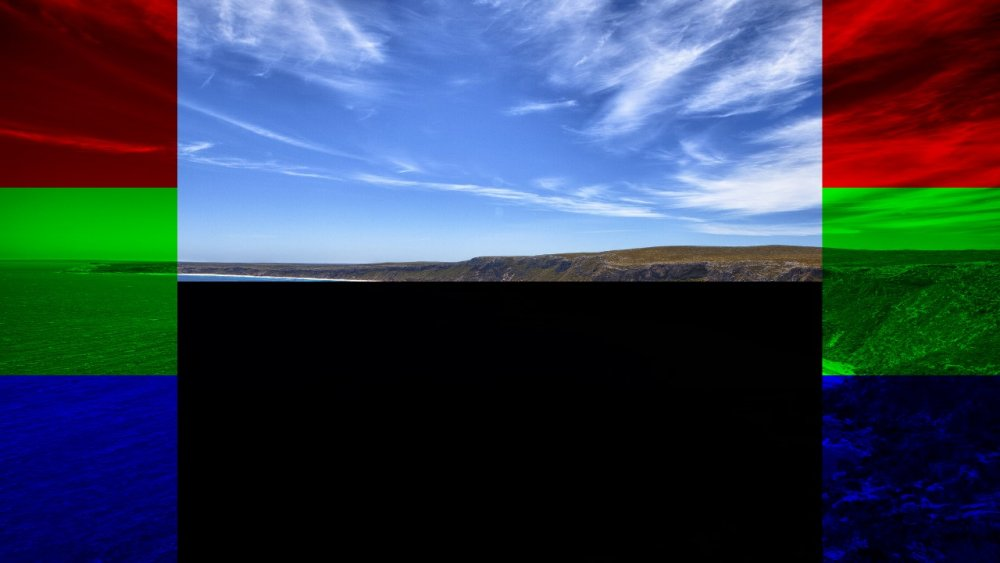Add Adds the values of image A and B together to produce a brighter image.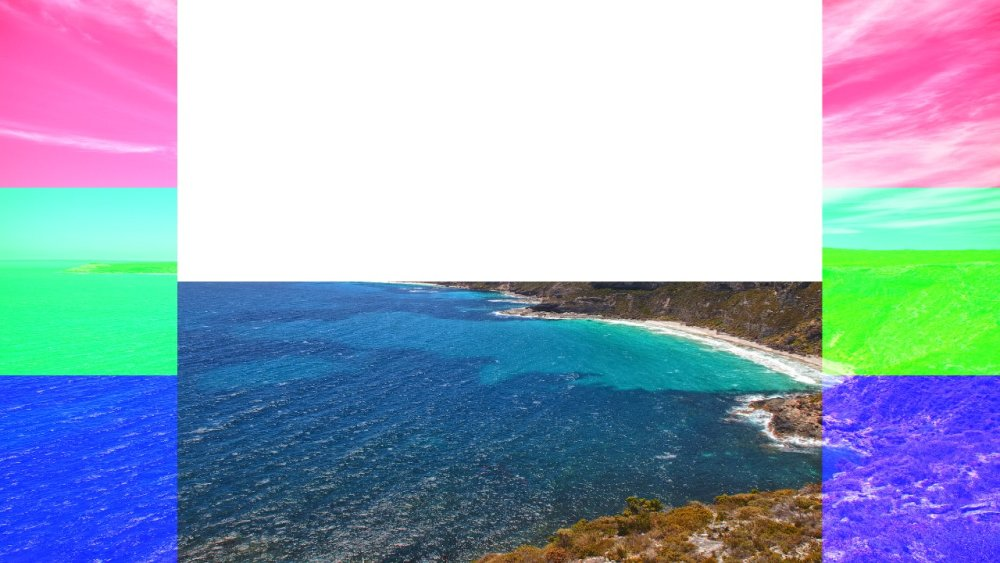Subtract Subtracts the values of image B from image A to produce a new image.Linear Image B is placed over Image A – however the alpha values are ignored.Max Image A and B are compared, and the maximum values are used.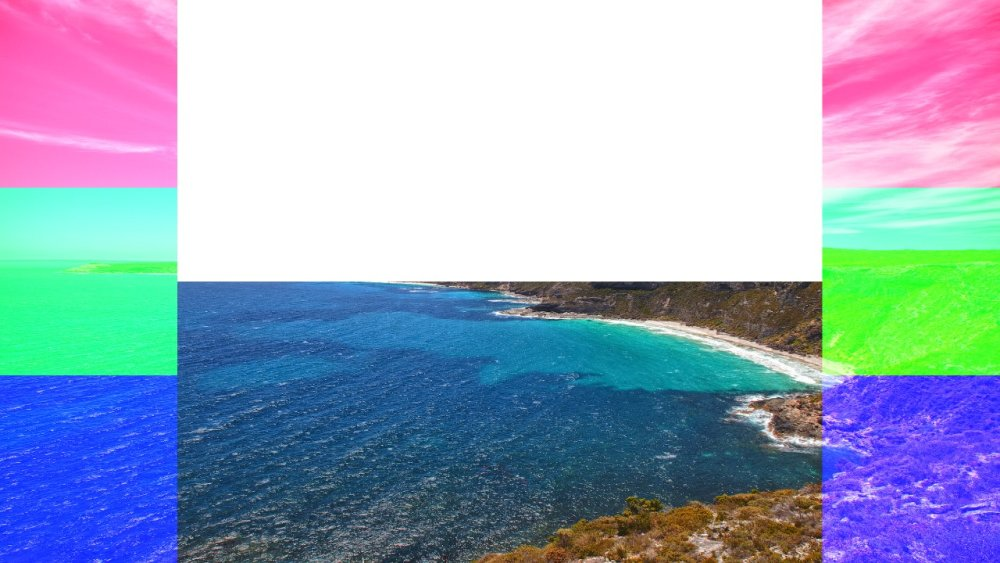Min Image A and B are compared, and the minimum values are used.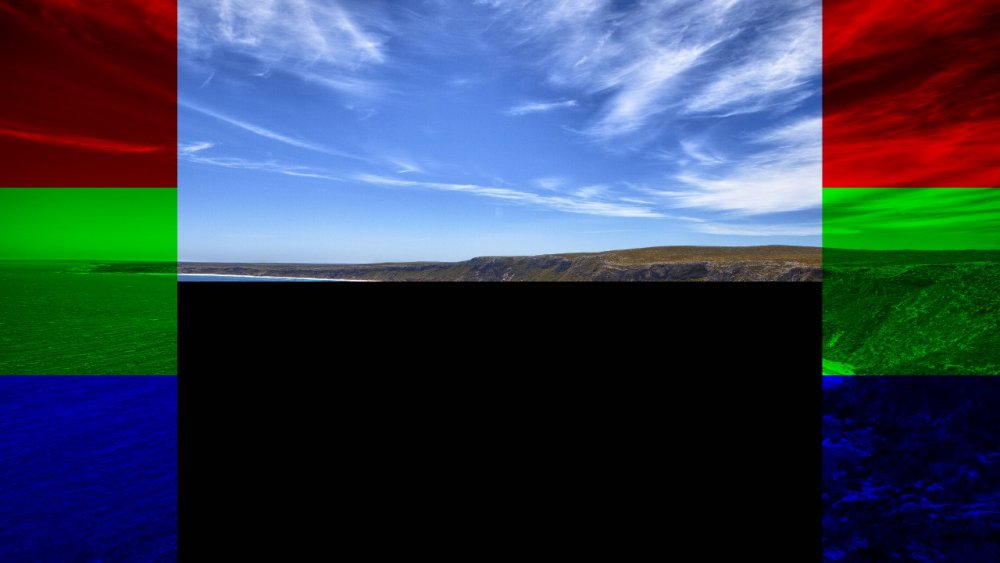Screen Image A and B are inverted, multiplied together and inverted again, producing a brighter image.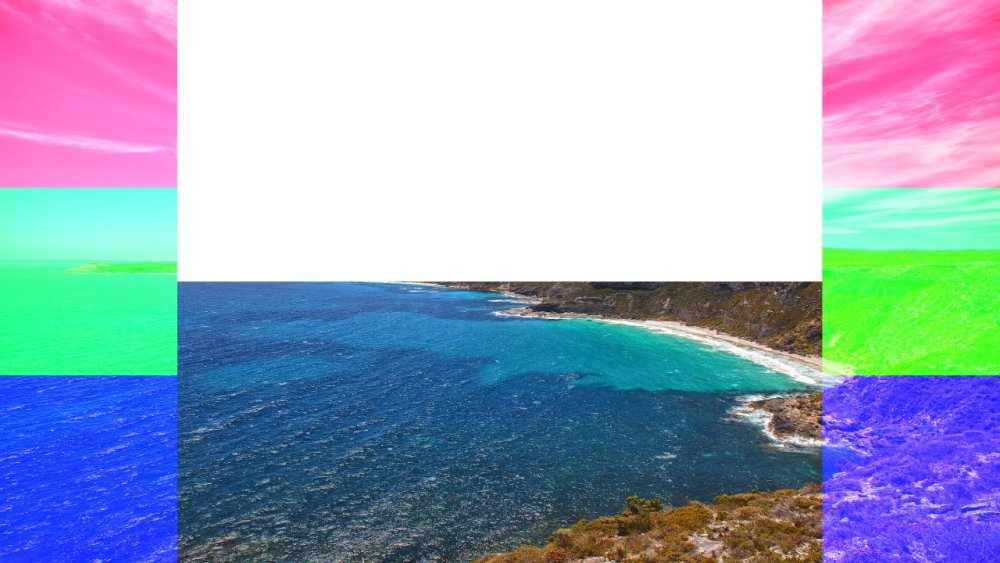Light Image A is multiplied by Image B, and then Image A is added again, resulting in a brighter image.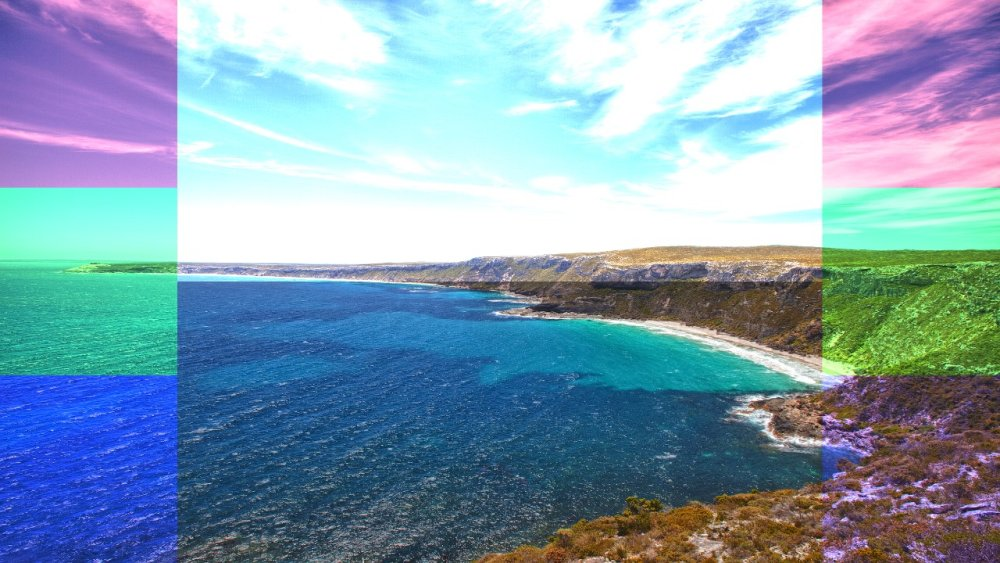Difference Image B is subtracted from Image A, and the absolute value is used to produce an image that highlights the differences between Image A and Image B. Only available within the Composite Sources node.Exclusion Image A and Image B are multiplied together and then by 2, then subtracted from Image A added to Image B. The result is very similar to Difference, but with lower contrast. Only available within the Composite Sources node.Dodge Image A is divided by the inverse of image B, producing an image that lightens Image A based on Image B. Only available within the Composite Sources node.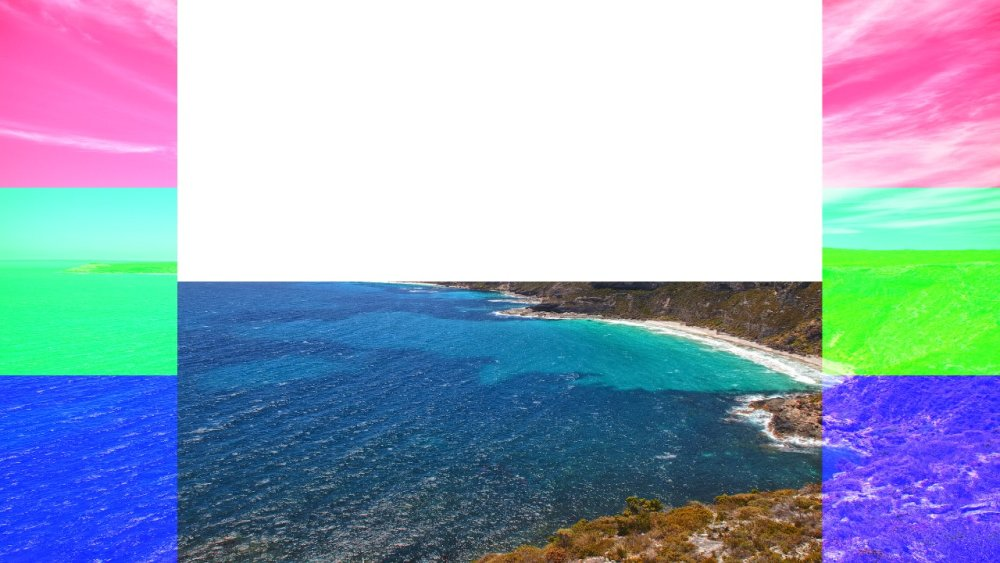Burn Image A is inverted, divided by image B and the result is inverted, producing an image that darkens Image A based on Image B. Only available within the Composite Sources node.Negate Image B is subtracted from image A, subtracted from 1, and the absolute value is subtracted from 1. This is the inverse of the difference blend mode. Only available within the Composite Sources node.